Question

Find an equation of the tangent plane to the surface 2 x4 = y2 +22 at...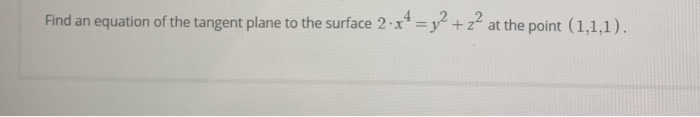Find an equation of the tangent plane to the surface 2 x4 = y2 +22 at the point (1,1,1).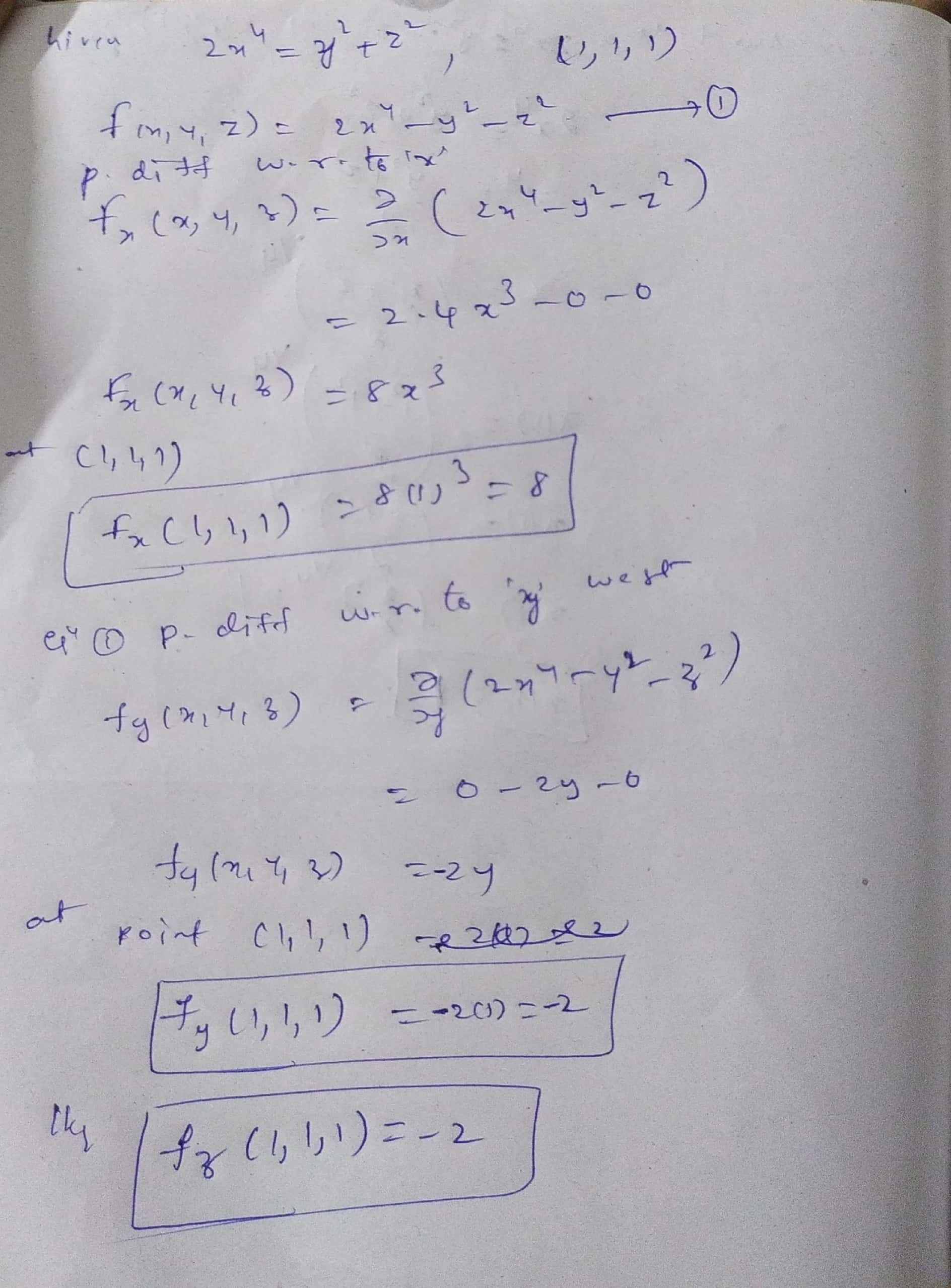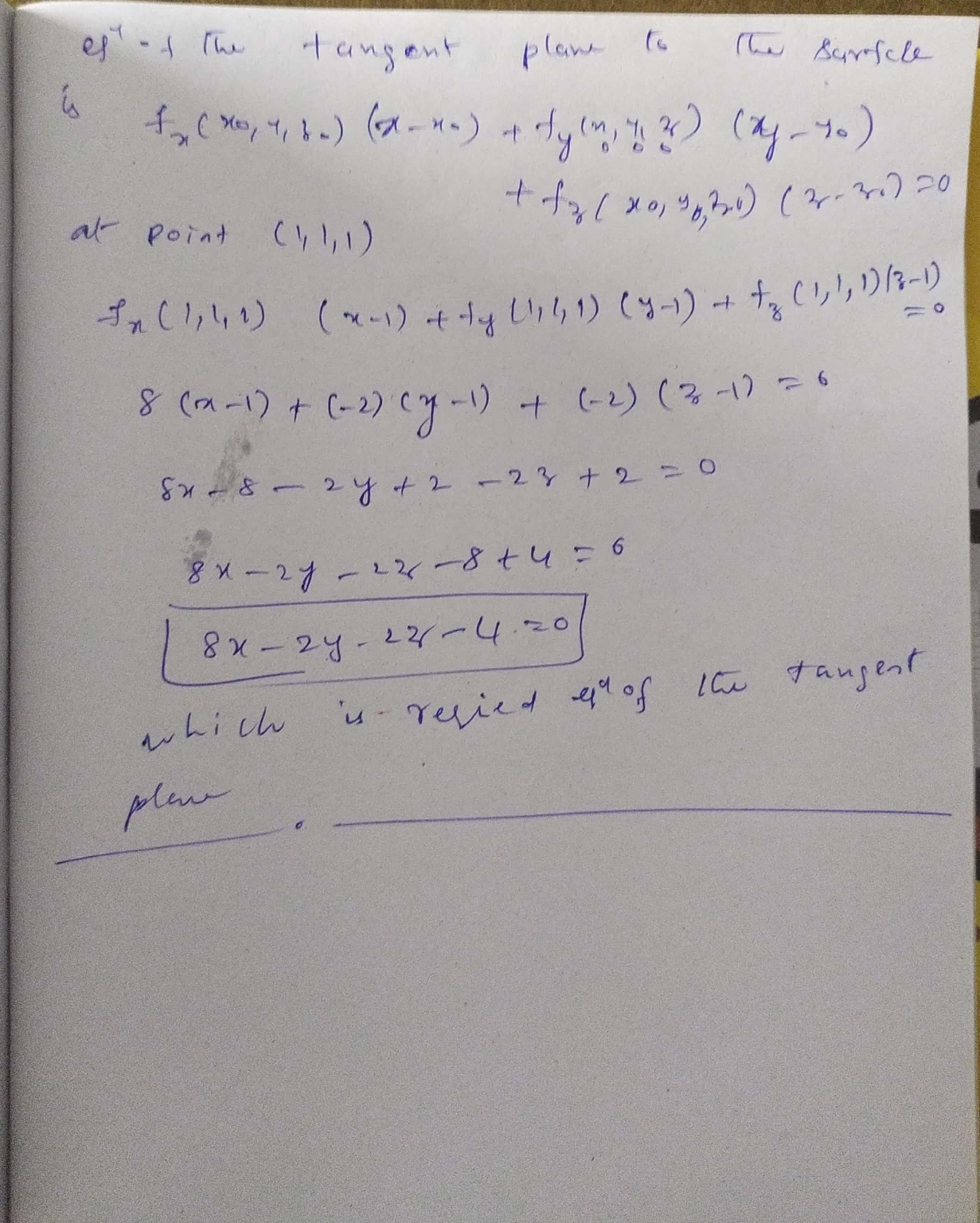Earn Coins

Coins can be redeemed for fabulous gifts.

Similar Homework Help Questions
• Partial derivatives Example Find the equation of the tangent plane and the normal line at the...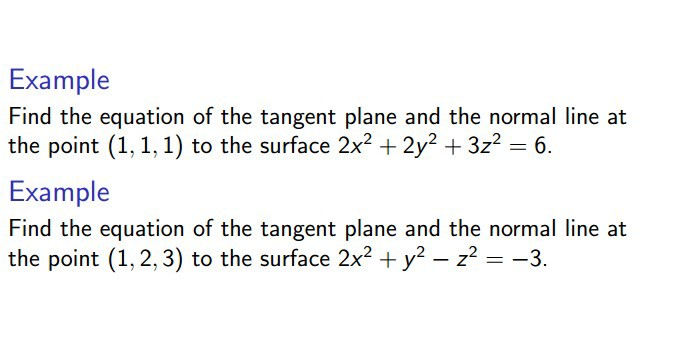Partial derivatives Example Find the equation of the tangent plane and the normal line at the point (1,1,1) to the surface 2x2 + 2y2 + 3z2 = 6. Example Find the equation of the tangent plane and the normal line at the point (1, 2, 3) to the surface 2x2 + y2 – z2 = -3.

• Find the equation of the tangent plane to the surface 2x2 + y2 + 2z2 =...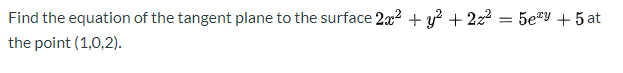Find the equation of the tangent plane to the surface 2x2 + y2 + 2z2 = 5ey + 5 at the point (1,0,2).

• Find the equation of the tangent plane to the surface at the given point a. z...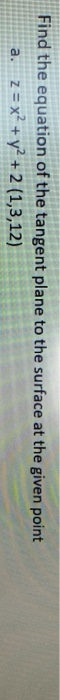Find the equation of the tangent plane to the surface at the given point a. z = x2 + y2 + 2 (1,3,12)

• Find the equation of the plane tangent to the following surface at the given points. x2...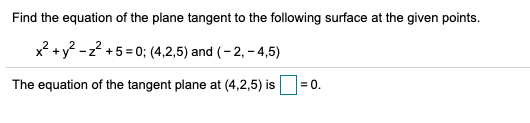Find the equation of the plane tangent to the following surface at the given points. x2 + y2 - 2? + 5 = 0; (4,2,5) and (-2,-4,5) The equation of the tangent plane at (4,2,5) is = 0. the equation of the tangent plane to the surface

• Find the equation for tangent plane and the normal line to the surface with equation x2...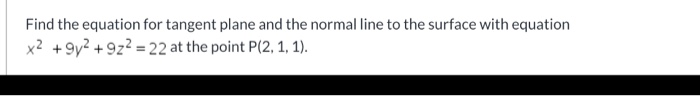Find the equation for tangent plane and the normal line to the surface with equation x2 +972 +922 = 22 at the point P(2, 1, 1).

• 6. Find an equation of the tangent plane to the surface z = 4x2-y2 +2y at...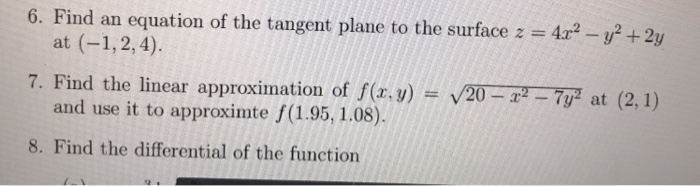6. Find an equation of the tangent plane to the surface z = 4x2-y2 +2y at (-1,2,4) = V20-下一77 at (2,1) 7. Find the linear approximation of f(z. y) and use it to approximte (1.95, 1.08). 8. Find the differential of the function

• Question 19 Find a normal vector of the tangent plane to 2? + y2 + x2...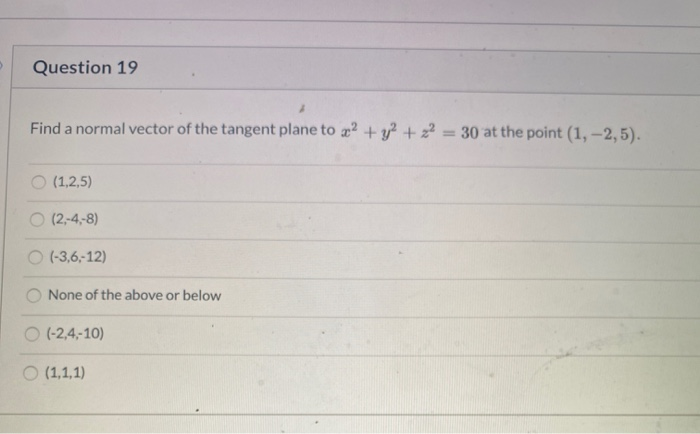Question 19 Find a normal vector of the tangent plane to 2? + y2 + x2 = 30 at the point (1, -2,5). (1,2,5) O (2,-4,-8) (-3,6,-12) None of the above or below (-2,4-10) (1,1,1)

• 1) Assume you are given the surface S with equation 2 1- (a) Find the equation of the tangent plane to S at the p...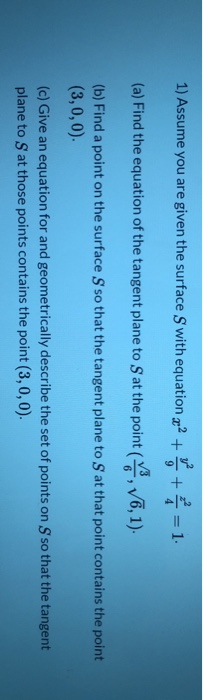1) Assume you are given the surface S with equation 2 1- (a) Find the equation of the tangent plane to S at the point (V6, 1) (b) Find a point on the surface S so that the tangent plane to S at that point contains the point (3,0, 0). (c) Give an equation for and geometrically describe the set of points on S so that the tangent plane to S at those points contains the point (3, 0,0). 1)...

• 2. DETAILS LARCALCETZM 13.7.021. (a) Find an equation of the tangent plane to the surface at...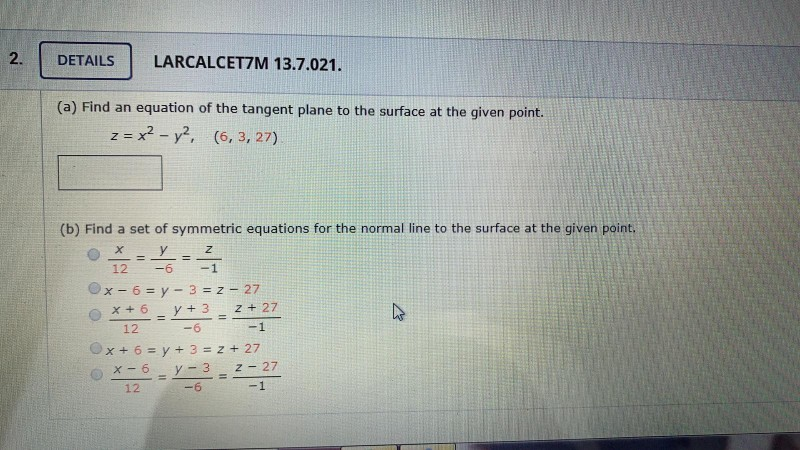2. DETAILS LARCALCETZM 13.7.021. (a) Find an equation of the tangent plane to the surface at the given point. z = x2 - y2, (6,3, 27) - 2 / 2 (b) Find a set of symmetric equations for the normal line to the surface at the given point. y = 2 12 -6 -1 x - 6 = y - 3 = 2 - 27 X + 6 y + 3 2 + 27 12 -6 -1 x + 6...

• 5. (2 points) Find the equation of the tangent plane to the given surface ation of...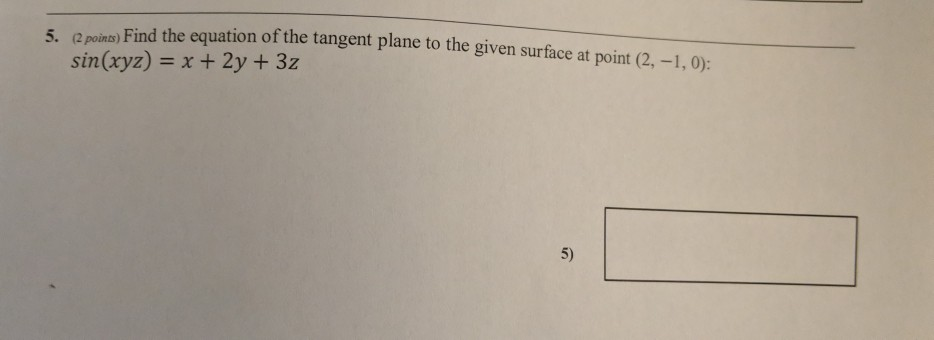5. (2 points) Find the equation of the tangent plane to the given surface ation of the tangent plane to the given surface at point (2. -1,0): sin(xyz) = x + 2y + 3z